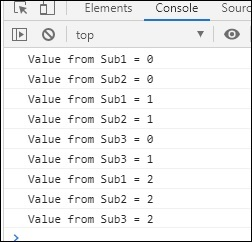# RxJS - Multicasting Operator publishReplay

publishReplay make use of behaviour subject, wherein, it can buffer the values and replay the same to the new subscribers and returns ConnectableObservable. The connect() method has to be used to subscribe to the observable created.

## Syntax

```publishReplay(value); // here value is the number of times it has to replay.
```

## Example

```import { interval} from 'rxjs';
import { take, publishReplay} from 'rxjs/operators';

let observer = interval(1000).pipe(
take(3),
publishReplay(2)
);
const subscribe_one = observer.subscribe(
x => console.log("Value from Sub1 = "+x)
);
const subscribe_two = observer.subscribe(
x => console.log("Value from Sub2 = "+x)
);
observer.connect();
setTimeout(() => {
const subscribe_three = observer.subscribe(
x => console.log("Value from Sub3 = "+x)
);
}, 2000);
```

## Output